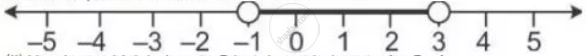Share

# Given a = {X: -1 < X ≤ 5, X ∈ R} and B = {X: -4 ≤ X < 3, X ∈ R} Represent on Different Number Lines: a ∩ B - Mathematics

Course

#### Question

Given A = {x: -1 < x ≤ 5, x ∈ R} and B = {x: -4 ≤ x < 3, x ∈ R}

Represent on different number lines:

A ∩ B

#### Solution

A ∩ B = {x: -1 < x < 3, x ∈ R}

it can be represented on a number line as:Is there an error in this question or solution?